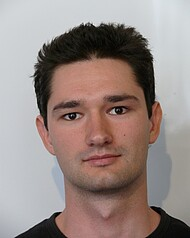Avoiding Root-Finding in the Krusell-Smith Algorithm Simulation | Munich Center for the Economics of Aging - MEA# Avoiding Root-Finding in the Krusell-Smith Algorithm Simulation

Inhalt

This paper proposes a novel method to compute the simulation part of the Krusell-Smith (1997, 1998) algorithm when the agents can trade in more than one asset (for example, capital and bonds). The Krusell-Smith algorithm is used to solve general equilibrium models with both aggregate and uninsurable idiosyncratic risk and can be used to solve bounded rationality equilibria and to approximate rational expectations equilibria. When applied to solve a model with more than one financial asset, in the simulation, the standard algorithm has to impose equilibria for each additional asset (find the market-clearing price), for each period simulated. This procedure entails root-finding for each period, which is computationally very expensive. I show that it is possible to avoid this rootfinding by not imposing the equilibria each period, but instead by simulating the model without market clearing. The method updates the law of motion for asset prices by using Newton-like methods (Broyden’s method) on the simulated excess demand, instead of imposing equilibrium for each period and running regressions on the clearing prices. Since the method avoids the root-finding for each time period simulated, it leads to a significant reduction in computation time. In the example model, the proposed version of the algorithm leads to a 32% decrease in computational time, even when measured conservatively. This method could be especially useful in computing asset pricing models (for example, models with risky and safe assets) with both aggregate and uninsurable idiosyncratic risk since methods which use linearization in the neighborhood of the aggregate steady state are considered to be less accurate than global solution methods for these particular types of models.

PublikationsdetailsIvo Bakota

2020
CERGE-EI Working Paper Series
online first